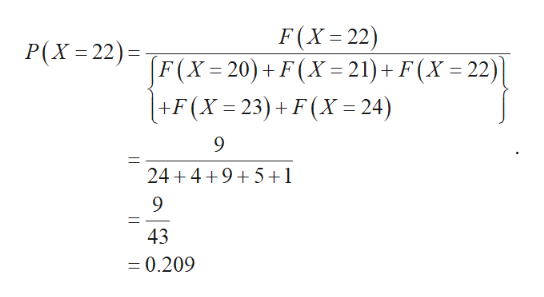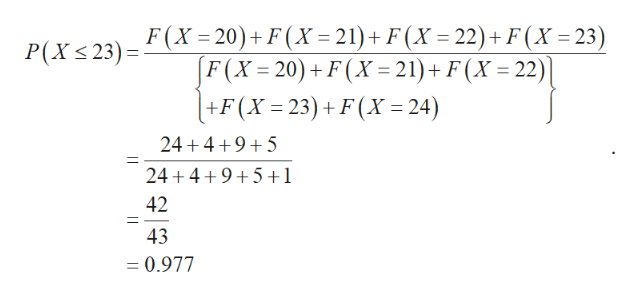# xx20202121222223232424F(x)F(x)242444995511Let xx be the ages of students in a class. Given the frequency distribution F(x)F(x)above, determine the following probabilities: (a) P(x=22)=P(x=22)=equation editorEquation Editor (b) P(x≤23)=P(x≤23)=equation editorEquation Editor (c) P(20≤x<22)=P(20≤x<22)=equation editorEquation Editor

Question
33 views

 xx 2020 2121 2222 2323 2424 F(x)F(x) 2424 44 99 55 11

Let xx be the ages of students in a class. Given the frequency distribution F(x)F(x)above, determine the following probabilities:

(a) P(x=22)=P(x=22)=

equation editor
Equation Editor

(b) P(x≤23)=P(x≤23)=

equation editor
Equation Editor

(c) P(20≤x<22)=P(20≤x<22)=

equation editor
Equation Editor
check_circle

Step 1

Here the numbers are repeated twice. So consider the below table for further calculations.

Step 2

(a)

The value of P(x=22) is obtained below:help_outlineImage TranscriptioncloseF(X 22) P(X=22)= F(X = 20) + F(X = 21)+F(X = 22) | - +F(X 23)+F(X= 24) 24 4 9 5+1 43 =0.209 fullscreen
Step 3

(b)

The value of P(x≤23) i...help_outlineImage TranscriptioncloseP(X23)=(X = 20)+ F(X=21) + F (X = 22)+ F(X = 23) F(X 20) F (X 21)+ F(X 22)] +F(X 23)+F(X = 24) 24 4 9 5 24 4 9 5+1 42 43 - 0.977 fullscreen

### Want to see the full answer?

See Solution

#### Want to see this answer and more?

Solutions are written by subject experts who are available 24/7. Questions are typically answered within 1 hour.*

See Solution
*Response times may vary by subject and question.
Tagged in

### Other